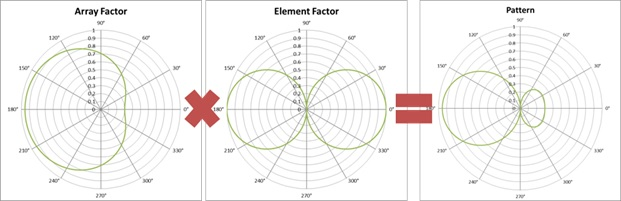# Loop Array Pattern CalculationsUsing an Excel spreadsheet, I have created an easy way to visualize my loop array pattern as I change frequency and phasing in the dual channel SDR.

So, I have an array of two wideband loops spaced 30 meters or 100 feet apart along a line roughly east-west. Is there any way I can visualize what the loop array pattern will be at various frequencies and phase adjustments? Yes, and here it is.

For a uniform linear array of two or more elements you have equal spacing between identical elements. In my case, N=2 (two elements) spaced 30 meters apart. Each element is identical – a wideband magnetic loop. My overall loop array pattern is a simple multiplication of the Array Factor times the Element Factor. The array factor assumes isotropic or point sources which radiate equally in all directions. The element factor is the actual radiation pattern of each antenna element.

You can draw your array factor using the array phase factor described previously. The formula is ψ = α + k d cos θ. Remember, α is the phasing you introduce in your receiver, and k d cosθ is the phasing introduced by element spacing, frequency and angle of arrival. The final array factor, normalized to 1, is calculated as follows:

Array Factor = sin(N ψ/2) ÷ [N sin(ψ/2)]

If you calculate the array factor for each degree from 0 to 360, you end up with the result shown above left. This calculation was made for 1530 kHz with 30 meter loop separation, end fire orientation and 90° phasing between channels in the SDR dual channel receiver. If you change the frequency, spacing or phasing in the SDR, the array factor changes accordingly. (Keep in mind we are considering only azimuth bearings. You can add elevation but that needs more math.)

## Loop Array Pattern Visualization

Your next step is the create the element factor, in this case the radiation pattern from a magnetic loop. Shown center above is the typical figure-8 pattern of a loop antenna along an E-W plane. You calculate this pattern using a math trick called the Bessel function.

Finally, when you multiply the array factor times the element factor, you get the loop array pattern shown above right. This pattern is great for receiving signals from the west and nulling stations from elsewhere. These calculations and visualizations required some mainly trig calculations in an Excel spreadsheet. I used the Polar Plot Excel add-in to create these graphs.

Using only 2 elements, either end fire or broadside, your results are mainly focused on creating nulls or reducing interference. You need at least four or more elements to do any fancy beamforming.

This site uses Akismet to reduce spam. Learn how your comment data is processed.